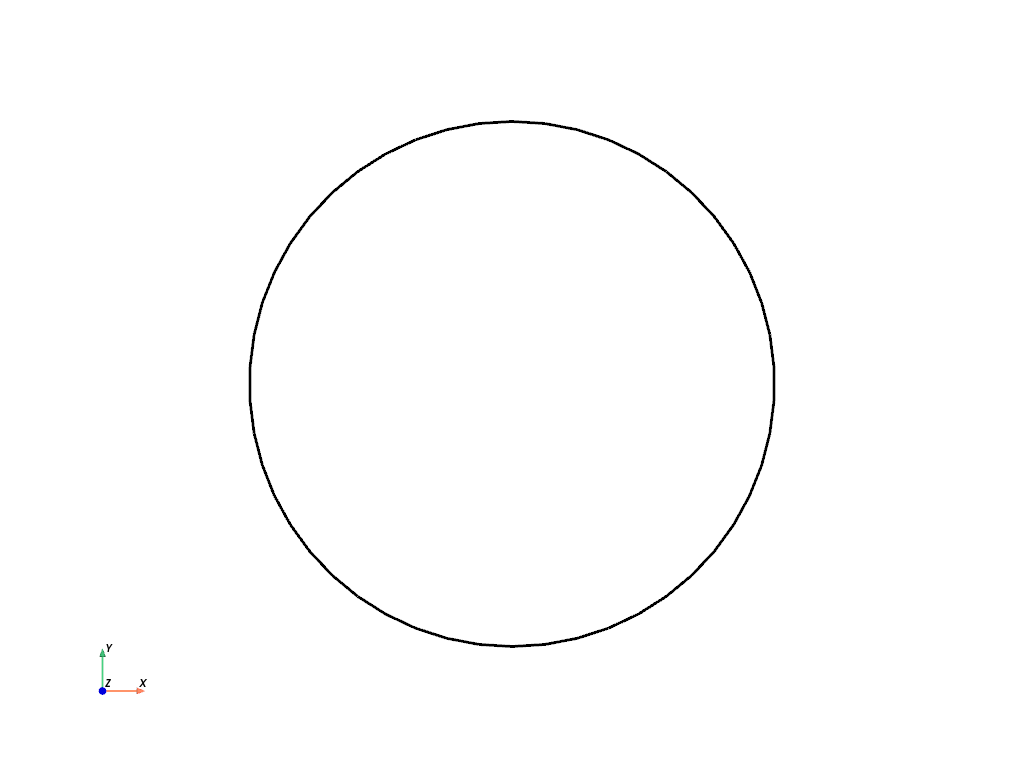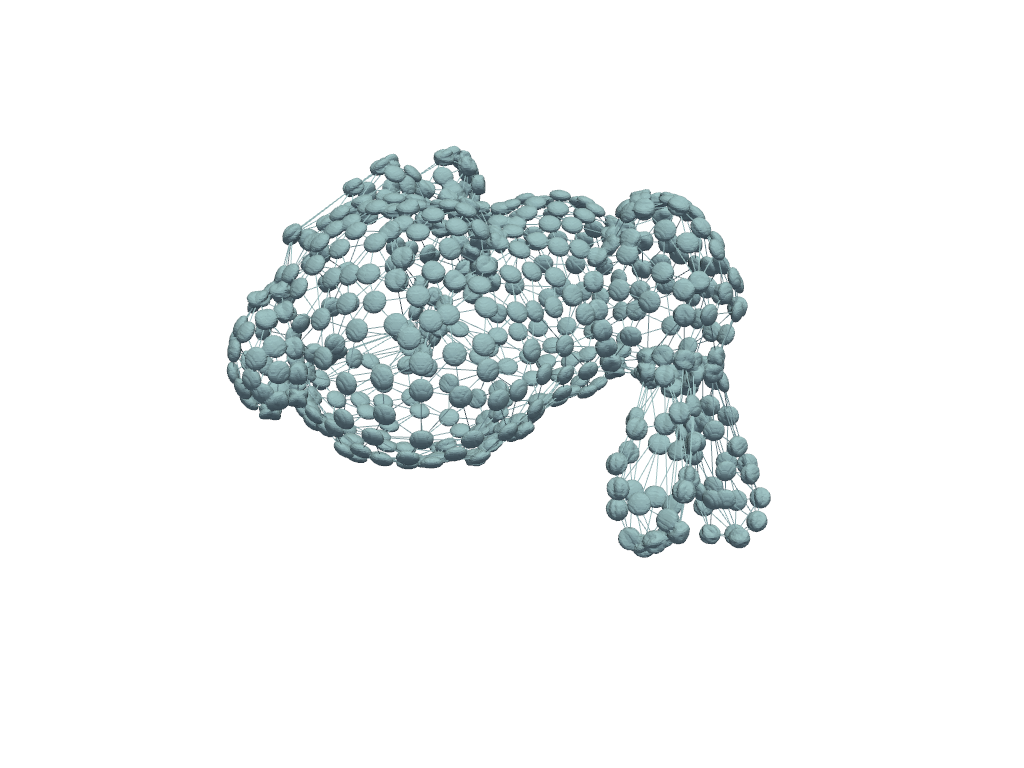# PyVista and VTK Together#

PyVista is best known for is easy to use plotting API – being familiar to most Python users already experienced with libraries like Matplotlib. Many people benefit from combining the power of VTK’s Python bindings for their data pipelines and the flexibility and simplicity of PyVista for 3D rendering. The following section demonstrates this usage scenario.

Tip

In case it was not made clear in the What is a Mesh? section, PyVista mesh classes are subclasses of their VTK counterparts - which means PyVista can be intermixed with VTK workflows.

Nothing stops you from using VTK classes and then wrapping the output with PyVista for streamlined plotting. For example:

```import pyvista as pv
from pyvista import examples
import vtk
```

Create a circle using vtk

```polygonSource = vtk.vtkRegularPolygonSource()
polygonSource.GeneratePolygonOff()
polygonSource.SetNumberOfSides(50)
polygonSource.SetCenter(0.0, 0.0, 0.0)
polygonSource.Update()
```

wrap and plot using pyvista

```mesh = pv.wrap(polygonSource.GetOutput())
mesh.plot(line_width=3, cpos='xy', color='k')
```In this manner, you can get the “best of both worlds” should you need the flexibility of PyVista and the raw power of VTK.

Note

You can use `pyvista.Polygon()` for a one line replacement of the above VTK code.

# VTK Algorithms#

Perhaps there is a VTK algorithm that is not (yet) exposed in PyVista that you’d like to use. This is easy enough to work with since PyVista objects are VTK objects. We can pass our PyVista meshes to the VTK algorithm, then wrap the output for plotting, further filtering, or anything.

```mesh = examples.download_bunny_coarse()
```

Initialize VTK algorithm

```splatter = vtk.vtkGaussianSplatter()
```

Pass PyVista object as input to VTK

```splatter.SetInputData(mesh)
```

Set parameters

```n = 200
splatter.SetSampleDimensions(n, n, n)
splatter.SetExponentFactor(-10)
splatter.SetEccentricity(2)
splatter.Update()
```

Retrieve output and wrap with PyVista

```vol = pv.wrap(splatter.GetOutput())
```

Use PyVista to produce contours

```cntrs = vol.contour([0.95 * splatter.GetRadius()])
```

Use PyVista to plot

```p = pv.Plotter()